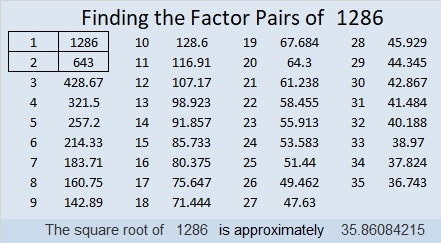# 1286 and Level 6

This level 6 puzzle can be solved by using logic and basic knowledge of the multiplication table. Stay focused, and you will get it done!Print the puzzles or type the solution in this excel file: 10-factors-1281-1288

Here are a few facts about the number 1286:

• 1286 is a composite number.
• Prime factorization: 1286 = 2 × 643
• The exponents in the prime factorization are 1 and 1. Adding one to each and multiplying we get (1 + 1)(1 + 1) = 2 × 2 = 4. Therefore 1286 has exactly 4 factors.
• Factors of 1286: 1, 2, 643, 1286
• Factor pairs: 1286 = 1 × 1286 or 2 × 643
• 1286 has no square factors that allow its square root to be simplified. √1286 ≈ 35.860841286 is also the sum of six consecutive prime numbers:
197 + 199 + 211 + 223 + 227 + 229 = 1286